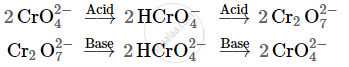Share

# Describe the Preparation of Potassium Dichromate from Iron Chromite Ore. What is the Effect of Increasing Ph on a Solution of Potassium Dichromate - Chemistry

ConceptSome Important Compounds of Transition Elements - Oxides and Oxoanions of Metals

#### Question

Describe the preparation of potassium dichromate from iron chromite ore. What is the effect of increasing pH on a solution of potassium dichromate?

#### Solution

Potassium dichromate is prepared from chromite ore (FeCr2O4) in the following steps.

Step (1): Preparation of sodium chromate

4FeCr_2O_4 + 16NaOH+7O_2 -> 8Na_2CrO_4+2Fe_2O_3+8H_2O

Step (2): Conversion of sodium chromate into sodium dichromate

2Na_2CrO_4 + conc.H_2SO_4 -> Na_2Cr_2O_7 + Na_2SO_4 + H_2O

Step(3): Conversion of sodium dichromate to potassium dichromate

Na_2Cr_2O_7 + 2KCl -> K_2Cr_2O_7 + 2NaCl

Potassium dichromate being less soluble than sodium chloride is obtained in the form of orange coloured crystals and can be removed by filtration.

The dichromate ion (Cr_2O_7^(2-)) exists in equilibrium with chromate (CrO_4^(2-)) ion at pH 4. However, by changing the pH, they can be interconverted.Is there an error in this question or solution?

#### Video TutorialsVIEW ALL 

Solution Describe the Preparation of Potassium Dichromate from Iron Chromite Ore. What is the Effect of Increasing Ph on a Solution of Potassium Dichromate Concept: Some Important Compounds of Transition Elements - Oxides and Oxoanions of Metals.
S